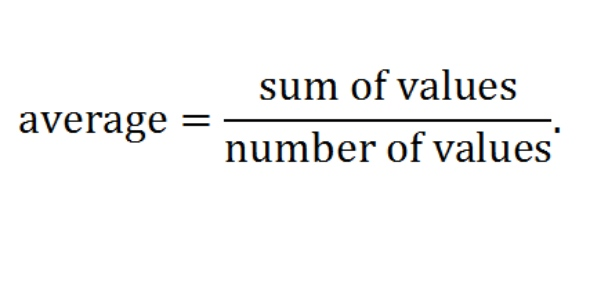What will be your formula for obtaining the average of the following - ProProfs DiscussTopicsMoreProductsMore+ Ask Question

# What will be your formula for obtaining the average of the following cells? A1=90 A2=85 A3=80 A4=75 A5=75Change Image    Delete

This question is part of MICROSOFT EXCEL EXAM
Asked by Wyatt Williams, Last updated: Feb 14, 2020

###Request 0FollowShareAnswer AnonymouslyAnswer LaterCopy Link#### A. Cook

Find happiness in writing new things.

A. Cook, English Professor, M.A, Ph.D, Kentucky

The formula to use in obtaining the average of the cells listed above in Microsoft excel is = average (a1:a5).
To get average, you add the values of all the items in the cells divided by the number of cells
= (a1 +a2+ a3 +a4+ a5)/ 5
Therefore, the two possible formulas of obtaining average in Microsoft excel are:
= average (a1:a5).
= (a1 +a2+ a3 +a4+ a5)/ 5
Hope this helps.=AVERAGE(A1:A2),=(A1+A2+A3+A4+A5)/5,=average(a1:a5),=(a1+a2+a3+a4+a5)/5#### engmaroof

Engmaroof

To get the average of range a1:a5 the formula should be
= average(a1:a5)#### londafb

Londafb

To average, you add the numeric values of all the items and then divide by the NUMBER OFITEMS added.
Capitalization is not an issue...

• =(A1+A2+A3+A4+A5)/5
• =AVERAGE(a1:a5)

I got the question right, but my answer was marked "wrong".#### wbonhart

Wbonhart

My answer was =average(a1:a5). I did not use uppercase letters. You do not have to use uppercase letters in Excel for the formula to work. So my answer is not wrong#### John Smith

John Smith=AVERAGE(A1:A5), =(A1+A2+A3+A4+A5)/5, =average(a1:a5), =(a1+a2+a3+a4+a5)/5Search for Google imagesSelect a recommended image
Upload from your computerCancelSearch for Google imagesSelect a recommended image
Upload from your computerCancelSearch for Google imagesSelect a recommended image
Upload from your computerCancel